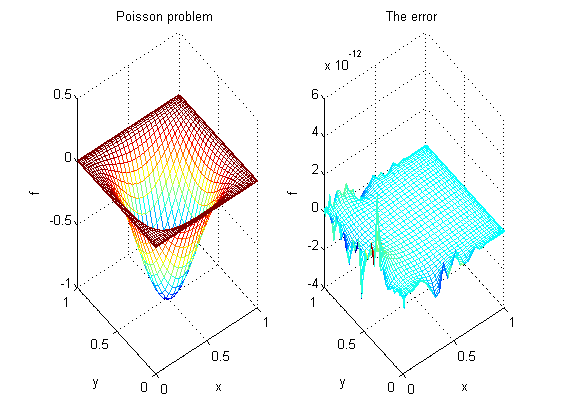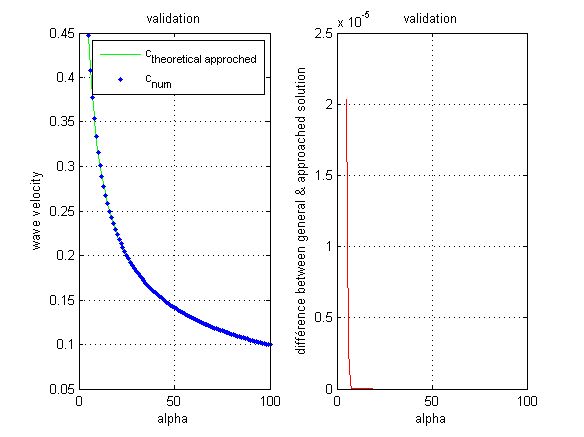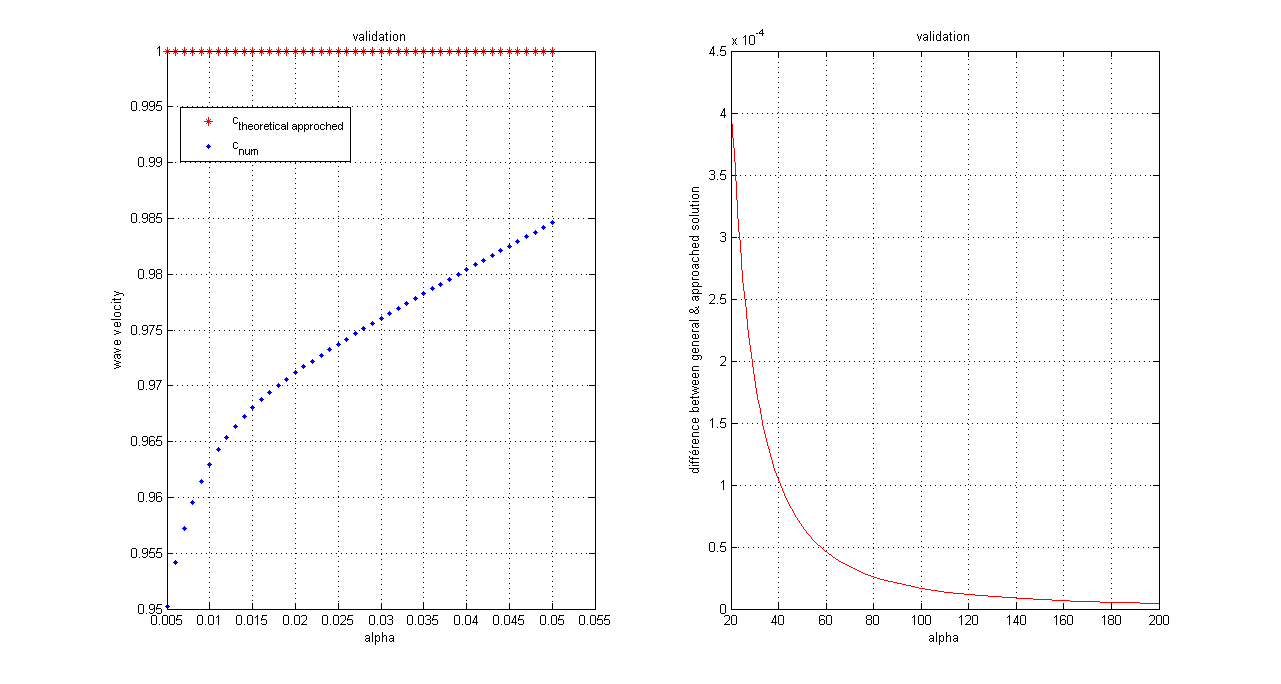# 1. Poisson problem in 2D: New validation case

Here we solve a Poisson problem in 2D with another forcing term to validate the first case.

### How to use the code with your own forcing term

When you have your forcing term, you will look for your new analytical solution.

When it’s done, you just have to modify:

• the forcing term in the section ‘New forcing case’ (you will change the second line ‘b = the_new_forcing_term’’)

• the new analytical solution in the section ‘plotting the result’ (so you will change ‘solexact’)

For example, we chose the forcing term:

\displaystyle b = -2\pi^2 cos(\pi k X) sin(\pi l Y)^2) sin(\pi l y)^2 - 2 \pi^2 cos(\pi k Y) sin(\pi l X)^2

and we found the exact solution :

\displaystyle f = sin(\pi X)^2 sin(\pi Y)^2

Here are the graphs for the analytical solution and for the error:# 2. Poisson problem in 2D: How to change the boundary conditions

### Dirichlet condition

For this problem, you have 4 borders alterable: the top, the bottom, the left, the right.

First of all, you must found the analytical solution of your problem and you must make sure that the continuity of the new function is verify (otherwise, you’ll have a singularity for the points concerned).

When you have your boundary condition, you will look for your new analytical solution.

When it’s done, you just have to modify:

• the new boundary condition in the section ‘boundary conditions’,for example, if you want to change the condition at the top, you will add the line ‘b(top)=f(x)’

• the new analytical solution in the section ‘plotting the result’ (so you will change ‘solexact’)

# 3. Free surface gravity

### 3.1 General solution

We showed in the general code that the wave velocity at the surface of the sea is:

\displaystyle c=\sqrt{\frac{g \tanh(\alpha L)}{\alpha}}

### 3.2 Short wavelengths

Short wavelengths solution

If we have a short wavelength comparared to the fluid depth, then we have:

\displaystyle \alpha L >> 1

so applying the Taylor expansion:

\displaystyle tanh(\alpha L)=1

so the wave velocity tends to :

\displaystyle c = \sqrt{g / \alpha}

This shows that the wave velocity depends on the wavenumber when this one become large: the system is dispersive.

Here are the graphs for the wave velocity as a fonction of the wave number. You can see :

• in the first one the graph of the numerical and the approached theoretically approached solution

• in the second one, the difference between the exact and the approached solutionwave velocity as a function of long wavenumbers \alpha

### 3.3 Long wavelength

If we have long wavelength compared to the fluid depth, then we have:

\displaystyle \alpha L << 1

so applying the Taylor expansion:

\displaystyle tanh(\alpha L)=\alpha L

so the wave velocity tends to :

\displaystyle c = \sqrt(g L)

This shows that the velocity of the waves does not depend on the wavelength when this one become large: the system is not dispersive.

Here are the graphs for the wave velocity as a fonction of the wave number. You can see :

• in the first one the graph of the numerical and the approached theoretically approached solution

• in the second one, the difference between the exact and the approached solutionwave velocity as a function of long wavenumbers \alpha

# Opening: futur contributions

I hope that the contributions were cleared. I started the theoretical and the numerical study of several wave cases like :

• the influency of the capillarity
• the case of two diffent fluids
• a very thin and viscous layer
• parallels with the Rayleigh-Taylor instability

De la part de Jérôme

domaine	        valeur	note
connectivité 	2	1
recyclage	2	2
graphiques	2	1
théories	4	1
Originalité	4	2
note /14	14	7

Ce que tu as fait est original, mais la présentation laisse grandement à désirer. Il n’y a pas d’image pour la validation sur ton code de Poisson (tu l’as mis sur ta page de contribution mais ce n’est pas là que c’est utile). Ton initiative était bonne de valider les grandes et petites longueurs d’onde mais ça ne marche pas pour les petites. Est-ce que tu as abandonné sans essayer de voir ce qu’il se passe. Tu n’explique même pas que ça ne marche pas, il faut que le lecteur le comprenne par lui-même. Ce n’est pas un travail de niveau M1…